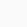# How to use union(∪) symbol in LaTeX?

SymbolUnion
Symbol typeMathematics
Package (requirement)No
ArgumentNo
Latex command\cup
ExampleS1 \cup S2 → S1 ∪ S2

Union symbol looks like the English letter U. Which is represented by the command \cup in LaTeX.

You need to use the bigunion symbol when adding the set of numbers n for which you need to use the command \bigcup

\documentclass{article}
\begin{document}
$a \cup b$
$S_1 \cup S_2$
$\bigcup_{i=1}^k F_i$
$\bigcup\limits_{i=1}^n S_i$
\end{document}

Output :Scroll to Top